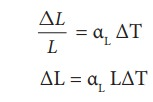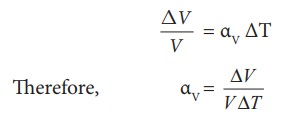Home | | Physics 11th std | Thermal expansion of solids, liquids and gases

# Thermal expansion of solids, liquids and gases

Thermal expansion is the tendency of matter to change in shape, area, and volume due to a change in temperature.

Thermal expansion of solids, liquids and gases

Thermal expansion is the tendency of matter to change in shape, area, and volume due to a change in temperature.

All three states of matter (solid, liquid and gas) expand when heated. When a solid is heated, its atoms vibrate with higher amplitude about their fixed points. The relative change in the size of solids is small. Railway tracks are given small gaps so that in the summer, the tracks expand and do not buckle. Railroad tracks and bridges have expansion joints to allow them to expand and contract freely with temperature changes. It is shown in Figure 8.3Liquids, have less intermolecular forces than solids and hence they expand more than solids. This is the principle behind the mercury thermometers.In the case of gas molecules, the intermolecular forces are almost negligible and hence they expand much more than solids. For example in hot air balloons when gas particles get heated, they expand and take up more space.

The increase in dimension of a body due to the increase in its temperature is called thermal expansion.

The expansion in length is called linear expansion. Similarly the expansion in area is termed as area expansion and the expansion in volume is termed as volume expansion. It is shown in Figure 8.4## Linear Expansion

In solids, for a small change in temperature ╬öT, the fractional change in length (ŌłåL/L) is directly proportional to ╬öT.Where, ╬▒L = coefficient of linear expansion.

╬öL = Change in length

L = Original length

╬öT = Change in temperature.

### EXAMPLE 8.6

Eiffel tower is made up of iron and its height is roughly 300 m. During winter season (January) in France the temperature is 2┬░C and in hot summer its average temperature 25┬░C. Calculate the change in height of Eiffel tower between summer and winter. The linear thermal expansion coefficient for iron ╬▒ = 10 ├Ś10ŌłÆ6 per ┬░C### Solution╬öL = 10 ├Ś 10ŌłÆ6 ├Ś 300 ├Ś 23 = 0.69 m=69 cm

## Area Expansion

For a small change in temperature ╬öT the fractional change in area (ŌłåA/A) of a substance is directly proportional to ╬öT and it can be written asWhere,

╬▒A = coefficient of area expansion.

╬öA = Change in area

A = Original area

╬öT = Change in temperature

## Volume Expansion

For a small change in temperature ╬öT the fractional change in volume (╬öV/V) of a substance is directly proportional to ╬öT.Where, ╬▒V = coefficient of volume expansion.

╬öV = Change in volume

V = Original volume

╬öT = Change in temperature

Unit of coefficient of linear, area and volumetric expansion of solids is ╦ÜC-1 or K-1

Study Material, Lecturing Notes, Assignment, Reference, Wiki description explanation, brief detail
11th Physics : UNIT 8 : Heat and Thermodynamics : Thermal expansion of solids, liquids and gases |